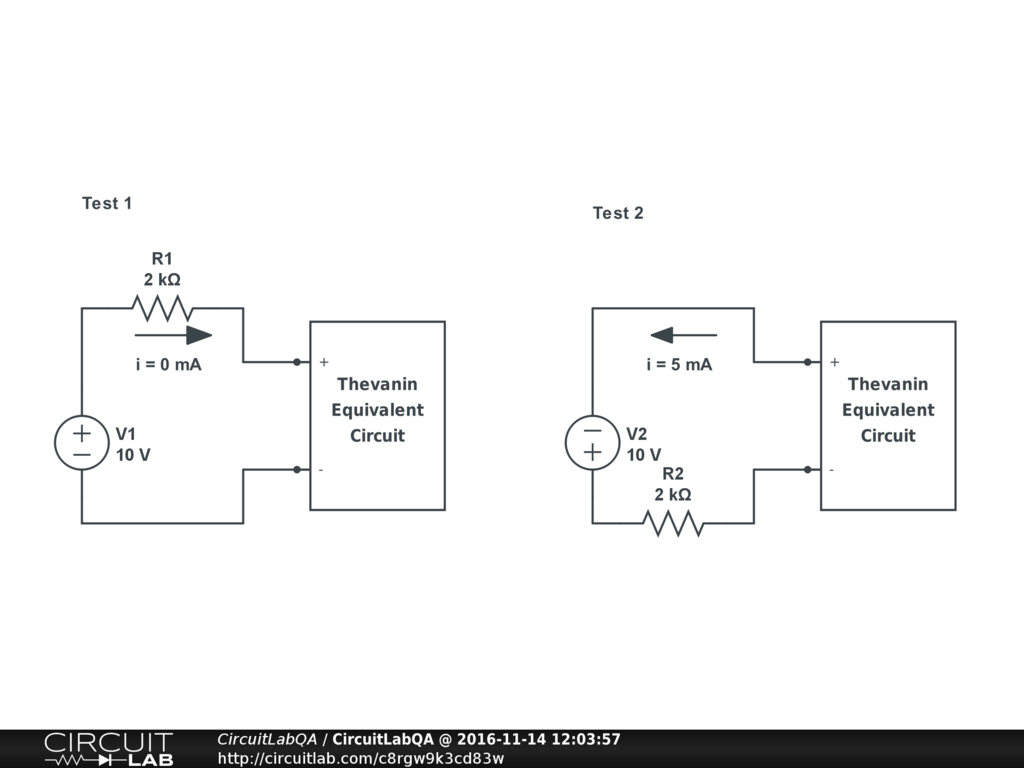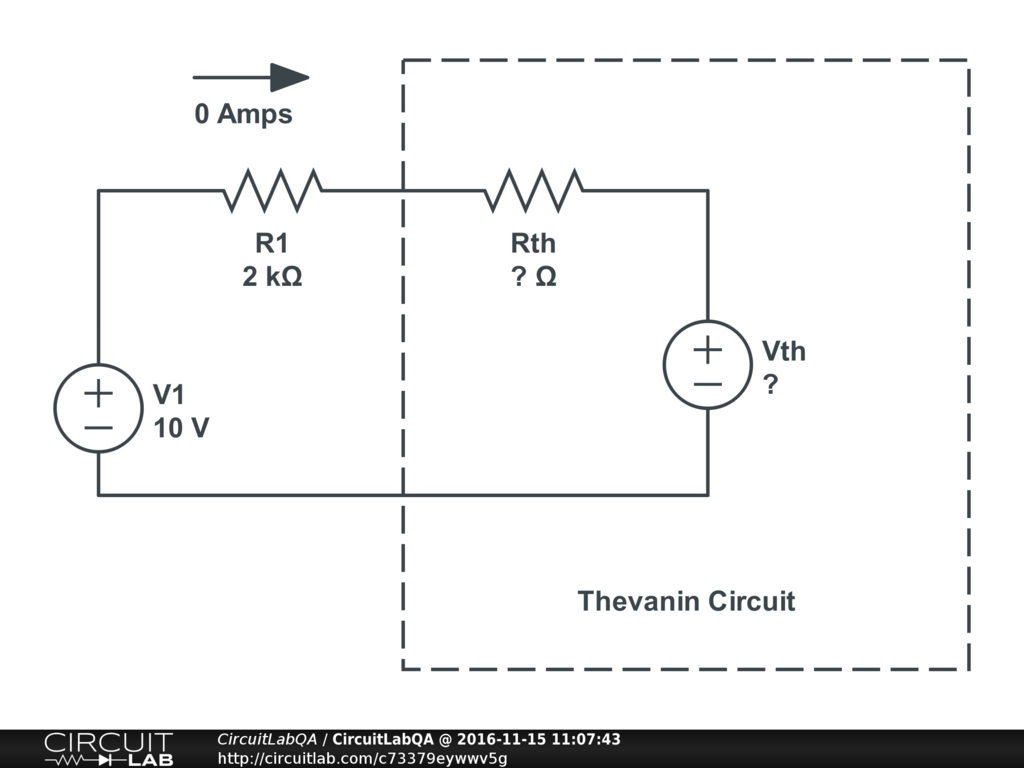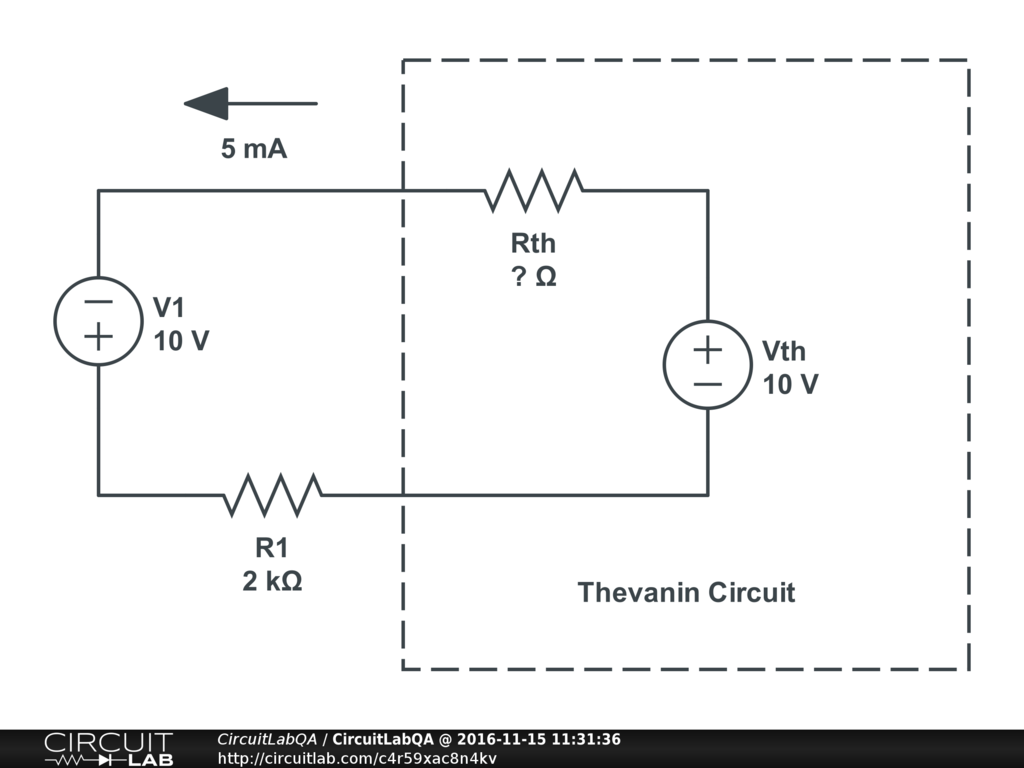## Thevanin equivalent circuit SOLVED

 I'm stuck on a question and I was wondering if somebody could help. This is supposed to be one Thevanin equivalent circuit, tested in two different ways. The measured current is shown in the diagram.I am having trouble figuring out how the current in the first test can be 0? by bmathre November 14, 2016

 Hi @bmarthe, This is a great question! Whenever doing thevanin equivalent problems I always like to draw the circuit out to see what I'm dealing with.Looking at this you can start to get an idea of how to answer your first question. The logic kind of goes like this: If 0 amps are going through R1, that means that 0 ams also going through R2. If no current is flowing through R1 + R2, then no voltage is dropped across those resistors. If no voltage is dropped across R1 + R2, then the positive terminal of $V_{th}$ has to be 10V. So if we fill in these values, and check out your second test case, we have thisLooking at the picture, we can make the following observations 5 mA must be going through $R_{th}$ 10V must be dropped across $R_{th}$ So from there its just a matter of plugging into $$V = IR$$ $$10V = 5mA \times R_{th}$$ $$R_{th} = \frac{10V}{5mA}$$ $$R_{th} = 2 \Omega$$ ACCEPTED +1 vote by hevans November 15, 2016

CircuitLab's Q&A site is a FREE questions and answers forum for electronics and electrical engineering students, hobbyists, and professionals.

We encourage you to use our built-in schematic & simulation software to add more detail to your questions and answers.

Acceptable Questions:

• Concept or theory questions
• Practical engineering questions
• “Homework” questions
• Software/hardware intersection
• Best practices
• Design choices & component selection
• Troubleshooting

Unacceptable Questions:

• Non-English language content
• Non-question discussion
• Non-electronics questions
• Vendor-specific topics
• Pure software questions
• CircuitLab software support

Please respect that there are both seasoned experts and total newbies here: please be nice, be constructive, and be specific!